Question

A 60 horsepower pump is used to move water from a large reservoir to another at a higher elevation. Measured data show...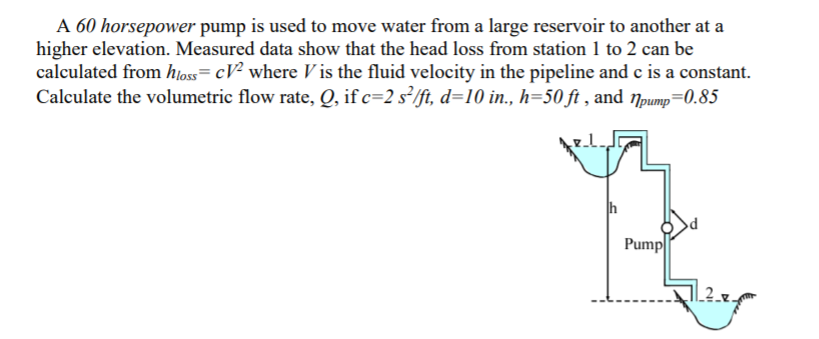A 60 horsepower pump is used to move water from a large reservoir to another at a higher elevation. Measured data show that the head loss from station 1 to 2 can be calculated from hloss= cV2 where V is the fluid velocity in the pipeline and c is a constant Calculate the volumetric flow rate, Q, if c=2 s2/ft, d=10 in., h=50 ft , and npump=0.85 h Pump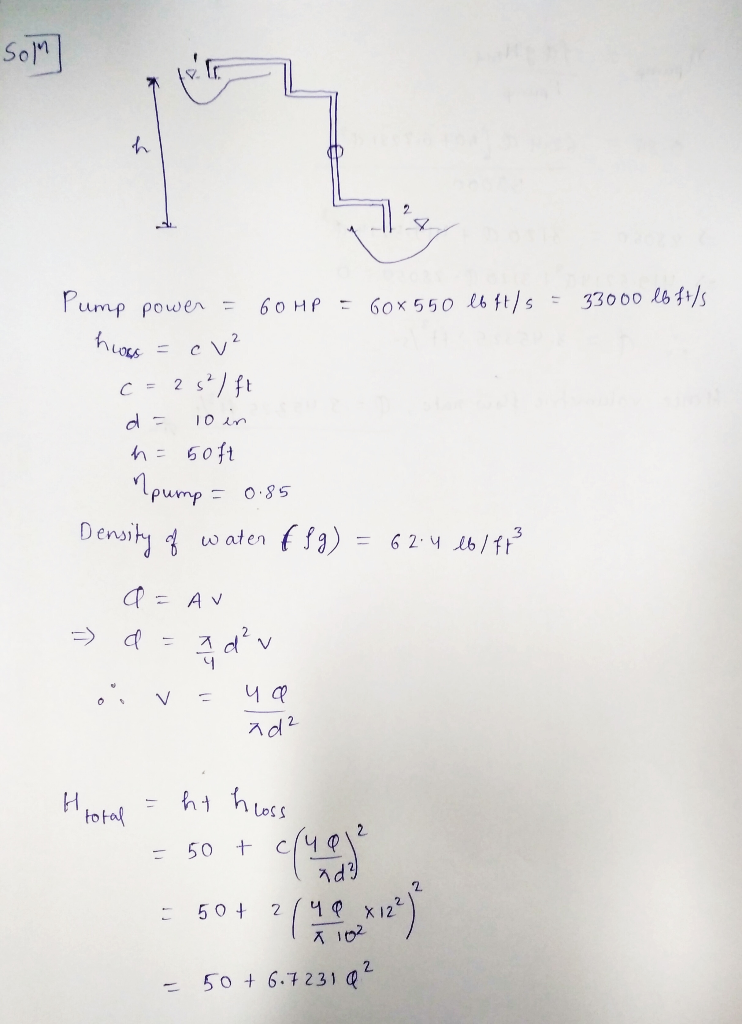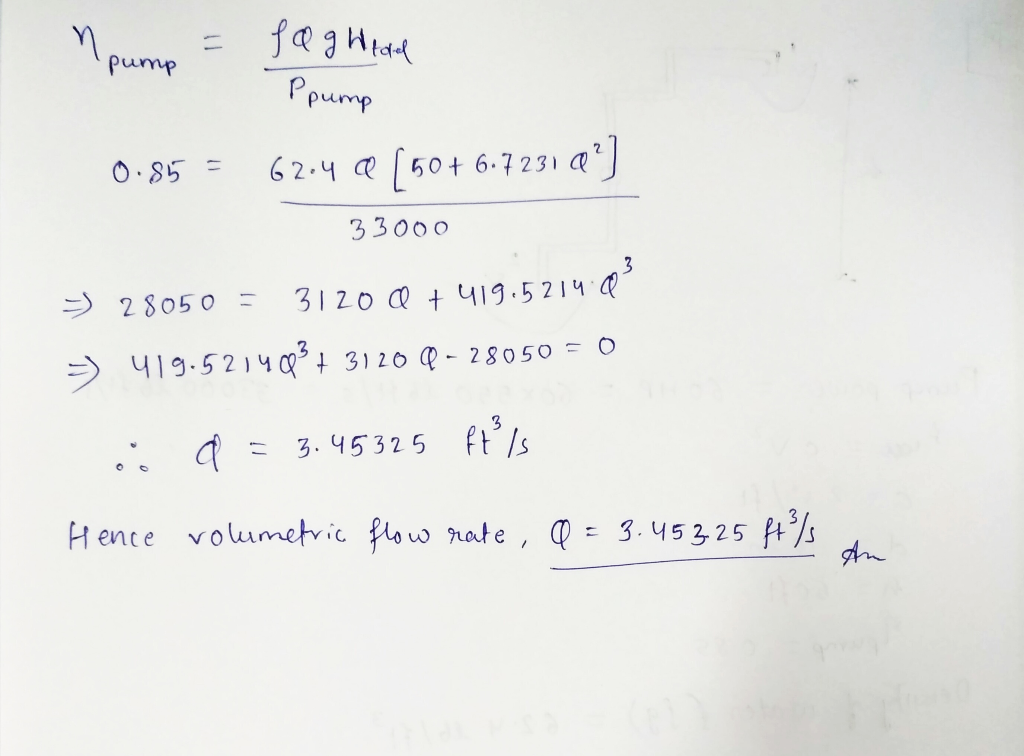Earn Coins

Coins can be redeemed for fabulous gifts.

Similar Homework Help Questions
• 4. Water is to be moved from one large reservoir to another a higher elevation as...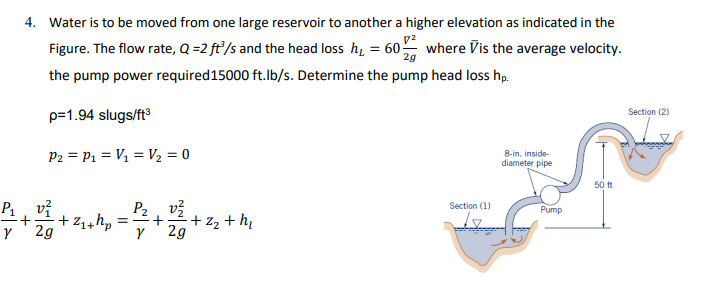4. Water is to be moved from one large reservoir to another a higher elevation as indicated in the Figure. The flow rate, Q=2 ft/s and the head loss hz = 60 where Vis the average velocity. the pump power required 15000 ft.lb/s. Determine the pump head loss hp. Section (2) p=1.94 slugs/ft3 P2 = P1 = V1 = V2 = 0 8-in. inside diameter pipe 50 Ft P2 + v +24thp = Section (1) Pump P2 vž ==+ +...

• 4. Water is to be moved from one large reservoir to another a higher elevation as...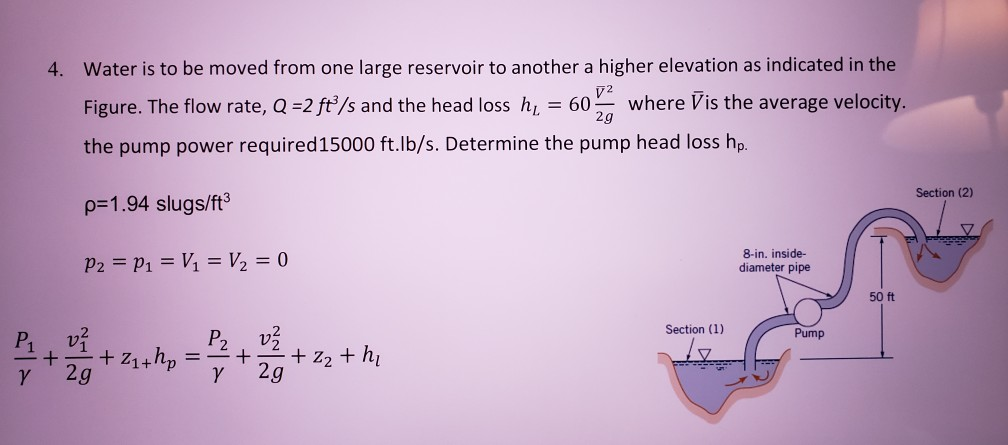4. Water is to be moved from one large reservoir to another a higher elevation as indicated in the V 2 Figure. The flow rate, Q =2 ft/s and the head loss hi = 60 where Vis the average velocity. 2g the pump power required 15000 ft.Ib/s. Determine the pump head loss hp. Section (2) p=1.94 slugs/ft P2 = P1 = V1 = V2 = 0 8-in. inside- diameter pipe 50 ft Section (1) Pump P v + + Z1+...

• A pump is used to deliver water from a large reservoir to another large reservoir that...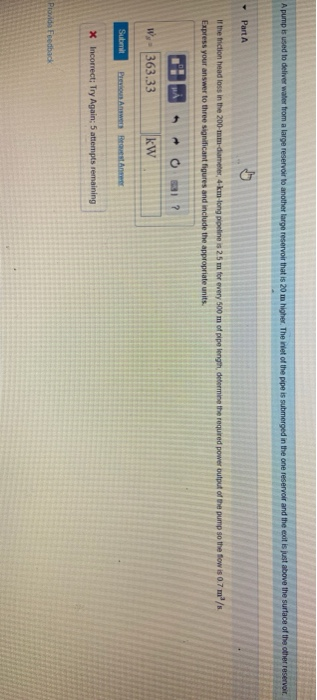A pump is used to deliver water from a large reservoir to another large reservoir that is 20 m higher. The net of the pipe is submerged in the one reservoir and the cat is just above the surface of the other reservoir Part A output of the pump so the fow is 0.7 m /s if the triction head loss in the 200-mm-diameter, 4-km-long pipeline is 25 m for every 500 m of pipe length determine Express your answer...

• 1 Award: 10.00 points used to pump water from one large reservoir to another large reservoir that is at a higher elevat...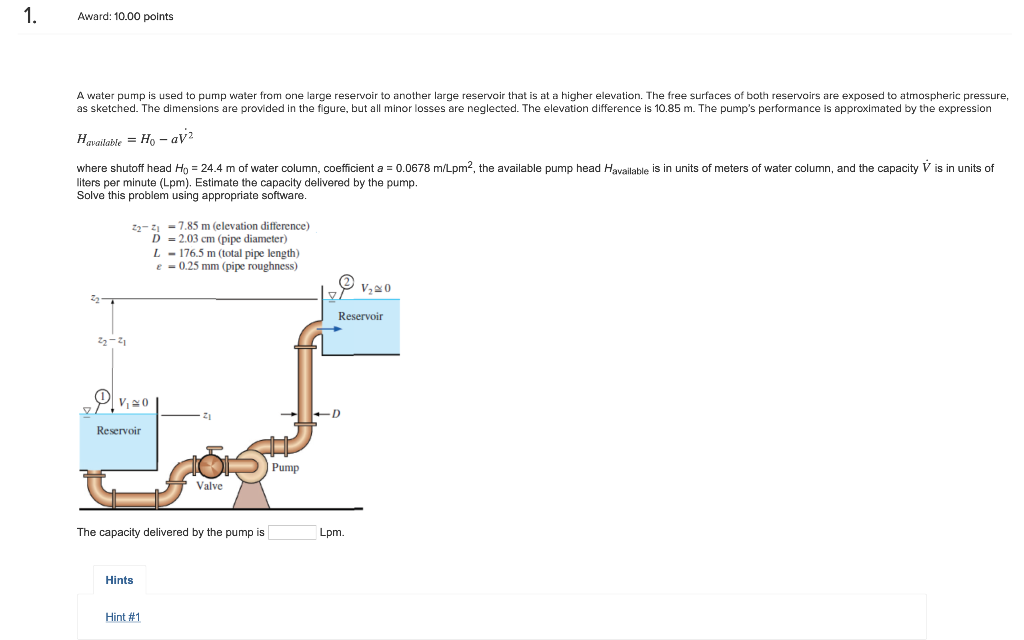1 Award: 10.00 points used to pump water from one large reservoir to another large reservoir that is at a higher elevation. The free surfaces of both reservoirs A water pump as sketched. The dimensions are provided in the fiqure, but all minor losses are neglected. The elevation difference is 10.85 m. The pump's performance is approximated by the expression exposed to atmospheric pressure Havailable = Ho- aV2 water column, coefficient a 0.0678 m/Lpm2, the available pump head Havailable is...

• Wp=Q×hp×gama Sec 729*24h 4. Water is to be moved from one large reservoir to another a...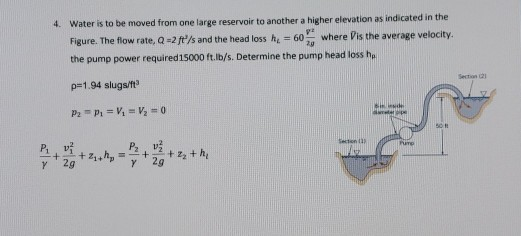Wp=Q×hp×gama Sec 729*24h 4. Water is to be moved from one large reservoir to another a higher elevation as indicated in the Figure. The flow rate, Q-2 ft/s and the head loss h = 60 where is the average velocity. 20 the pump power required 15000 ft.ib/s. Determine the pump head loss he p=1.94 slugs/ft P2 - P. = V = V2 = 0 Pit + + 2 thi Y SIR Sen PUTO

• NO QUESTIONS DURING T Water is to be pumped from a reservoir (ZA30 /s- to another reservoir at a higher elevati rate...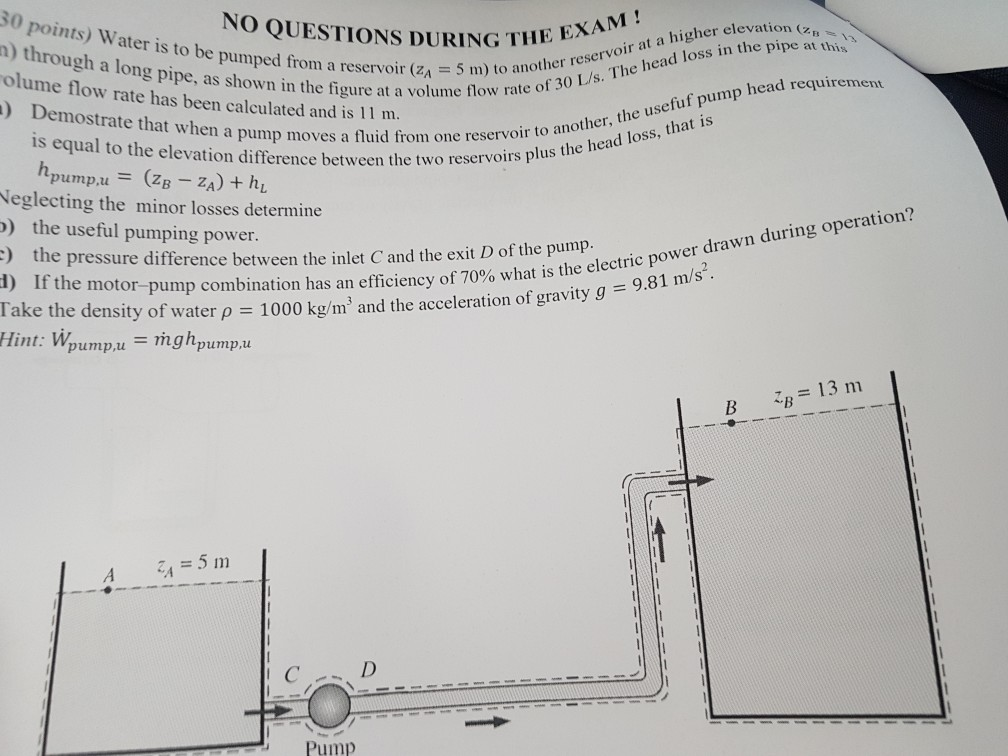NO QUESTIONS DURING T Water is to be pumped from a reservoir (ZA30 /s- to another reservoir at a higher elevati rate of elevation (Zn THE EXAM! n) through a long pipe, as shown in the figure at a volume ow olume flow rate has been calculated and is 11 m he head loss in the pipe at this usefuf pump ) Demostrate that wh en a pump moves a fluid from one reservoir to ano e elevation difference between...

• 14-34 A water pump is used to pump water from one large reservoir to another large...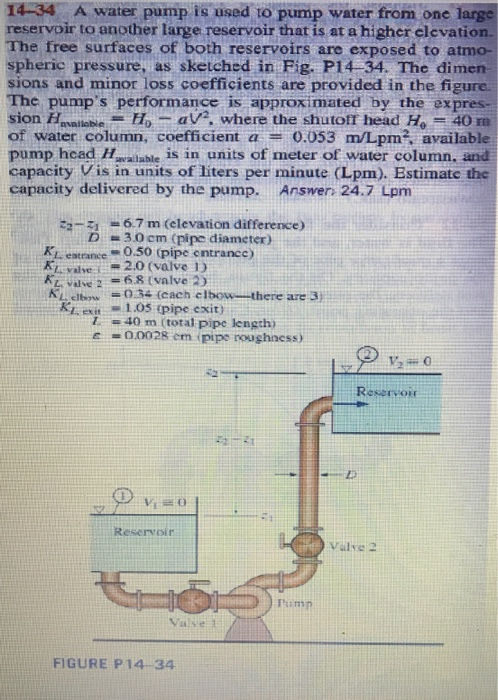14-34 A water pump is used to pump water from one large reservoir to another large reservoir that is at a higher elevation. The free surfaces of both reservoirs are exposed to atmo- spheric pressure, as sketched in Fig. P14-34. The dimen sions and minor loss coefficients are provided in the figure. The pump's performance is approximated by the expres- sion Havailable H av?, where the shutoff head H 40 m of water column, coefficient a = 0.053 m/Lpm?, available...

• Q4. A pump draws water from a large reservoir and delivers it to another reservoir whose water surface is 10 m higher than the pump centreline and that the pump is 1.5 meter above the suction reservo...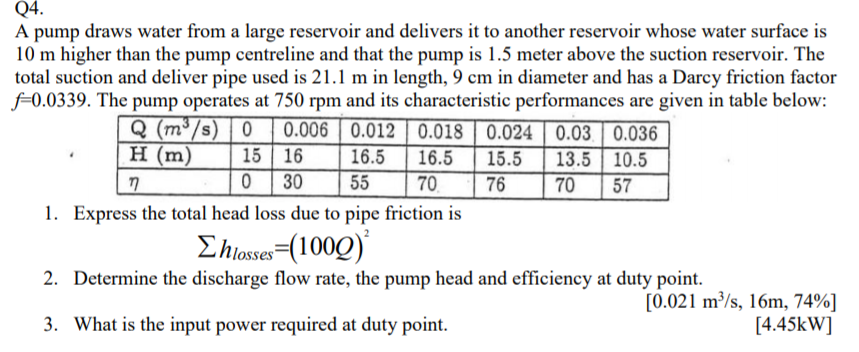Q4. A pump draws water from a large reservoir and delivers it to another reservoir whose water surface is 10 m higher than the pump centreline and that the pump is 1.5 meter above the suction reservoir. The total suction and deliver pipe used is 21.1 m in length, 9 cm in diameter and has a Darcy friction factor f0.0339. The pump operates at 750 rpm and its characteristic performances are given in table below: Q (m3 s H (m)15...

• Task 1: You were asked to check up the status of the water system. Water at 24 °C is to be pumped from a Lower reservoir with 100 m elevation to another reservoir at a higher elevation 112 m through...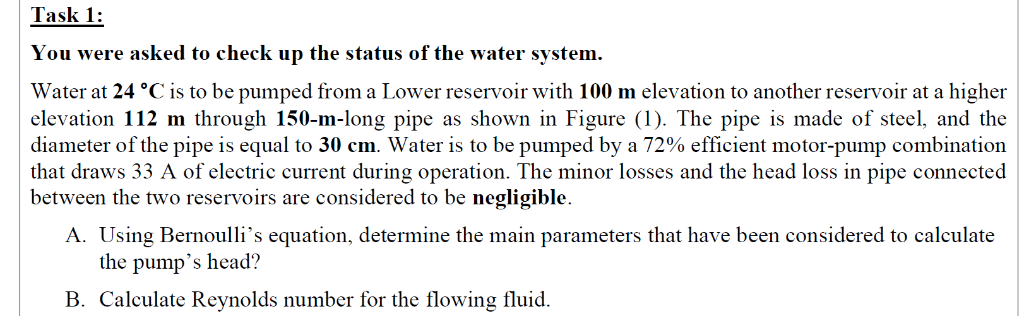Task 1: You were asked to check up the status of the water system. Water at 24 °C is to be pumped from a Lower reservoir with 100 m elevation to another reservoir at a higher elevation 112 m through 150-m-long pipe as shown in Figure (1). The pipe is made of steel, and the diameter of the pipe is equal to 30 cm. Water is to be pumped by a 72% efficient motor-pump combination that draws 33 A of...

• 11. Water is pumped from a reservoir at a lower elevation to an elevated reservoir by...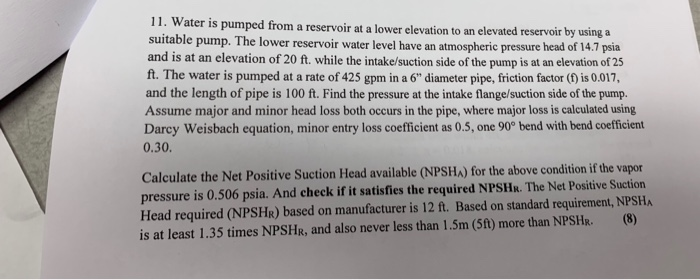11. Water is pumped from a reservoir at a lower elevation to an elevated reservoir by using a suitable pump. The lower reservoir water level have an atmospheric pressure head of I and is at an elevation of 20 ft. while the intake/suction side of the pump is at an elevation of 25 ft. The water is pumped at a rate of 425 gpm in a 6" diameter pipe, friction factor () is 0.017 and the length of pipe is...